Power Torque continues to be a leading integrator in the motion control and electrical / mechanical power transmission fields. Let our combined experience of over 400 years design a solution for you.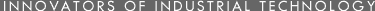• •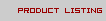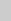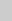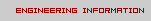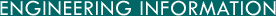S T U V W X Y Z

S

• Sack
= 3 bushels, 0.10911 cubic meter.

• Scruple (apothecary)
= 0.002857143 avoirdupois pound, 0.003472222 apothecary or troy pound, 0.0457143 avoirdupois ounce, 0.041667 apothecary or troy ounce, 0.7314286 avoirdupois dram, 1/3 apothecary or troy dram, 0.833333 pennyweight, 20 grains, 1.2959784 grams, 1295.9784 milligrams.

• Seam (Br)
= 8 bushels, 0.29095 cubic meter.

• Second (angle)

• Second (time, mean solar)
= 1.1574x10^-5 mean solar day, 1.1606x10^-5 sidereal day, 2.7778x10^-4 mean solar hour, 0.016667 mean solar minute, 1.00273791 sidereal seconds.

• Second (time, sidereal)
= 0.997270 mean solar second.

• Sign
= 30 degrees.

• Skein
= 360 feet, 120 yards, 109.73 meters.

• Slug
= 1 geepound, 32.174 pounds, 14.594 kilograms.

• Space, Entire (solid angle)

• Spherical Right Angle
= 0.125 sphere, 0.25 hemisphere, Pi/2 steradians.

• Square Centimeter
= 1x10^24 barns, 2.47104x10^-7 square chain, 3.95367x10^-6 square rod, 1.1960x10^-4 square yard, 0.0010764 square foot, 0.00247104 square link, 0.15500 square inch, 1.5500x10^5 square mils, 1.9735x10^5 circular mils, 127.32 circular millimeters, 1x10^-4 square meter, 0.01 square decimeter, 100 square millimeters.

• Square Chain (Gunter's)
= 1.5625x10-4 square mile, 16 square rods, 484 square yards, 4356 square feet, 1x10^4 square links, 6.27264x10^5 square inches, 404.6873 square meters.

• Square Decimeter
= 15500 square inch, 0.01 square meter, 100 square centimeters.

• Square Degree

• Square Dekameter
= 0.02471044 acre, 119.60 square yards, 1 are, 100 square meters.

• Square Foot
= 3.58701x10^-8 square mile, 2.29568x10^-5 acre, 2.29568x10^-4 square chain, 0.00367309 square rod, 1/9 square yard, 2.29568 square links, 144 square inches, 9.290341x10^-4 are, 0.09290341 square meter, 929.0341 square centimeters.

• Square Foot (Br)
= 0.90290289 square meter.

• Square Hectometer
= 2.471044 acres, 2.471058 acres (Br), 1x10^4 square meters.

• Square Inch
= 1.59423x10^-6 square chain, 1/144 square foot, 1/1296 square yard, 0.0159423 square link, 1x10^6 square mils, 1.27324x10^6 circular mils, 6.4516258x10^-4 square meter, 6.4516258 square centimeters, 645.16258 square millimeters.

• Square Kilometer
= 0.3861006 square mile, 247.1044 acres, 247.1058 Br acres, 1.1960x10^6 square yards, 1.0764x10^7 square feet, 1x10^6 square meters.

= 1x10^-5 acre, 1x10^-4 square chain, 0.0016 square rod, 0.0484 square yard, 0.4356 square foot, 62.7264 square inches, 0.040469 square meter, 404.69 square centimeters.

• Square Meter
= 3.8610x10^-7 square mile, 2.471044x10^-4 acre, 2.471058x10^-4 Br acre, 0.00247104 square Gunter's chain, 0.039537 square rod, 1.195985 square yards, 1.195992 square Br yards, 10.76387 square feet, 10.76390 square Br feet, 24.7104 square Gunter's links, 1550.0 square inches, 1x10^-6 square kilometer, 1x10^-4 hectare, 0.01 are, 1 centare, 1x10^4 square centimeters, 1x10^6 square millimeters.

• Square Mil
= 1x10^-6 square inch, 1.2732 circular mils, 6.4516x10^-6 square centimeter, 6.4516x10^-4 square millimeter.

• Square Mile
= 640 acres, 6400 square chains, 1.02400x10^5 square rods, 3.0976x10^6 square yards, 2.78784x10^7 square feet, 2.589998 square kilometers, 258.9998 hectares, 2.589998x10^6 square meters.

• Square Millimeter
= 0.0015500 square inch, 1550.0 square mils, 1973.5 circular mils, 1x10^-6 square meter, 0.01 square centimeter, 1.2732 circular millimeters.

• Square Perch
= 1/160 acre, 30.25 square yards, 25.293 square meters.

• Square Pole (Br)
= 30.25 square yards.

• Square Rod
= 9.765625x10^-6 square mile, 0.00625 acre, 0.0625 square Gunter's chain, 30.25 square yards, 272.25 square feet, 625 square Gunter's links, 3.9204x10^4 square inches, 0.0025293 hectare, 25.293 square meters.

• Square Yard
= 3.22831x10^-7 square mile, 2.06612x10^-4 acre, 0.00206612 square chain, 0.0330579 square rod, 9 square feet, 20.6612 square link, 1296 square inches, 8.36131x10^-5 hectare, 0.0083613 square dekameter, 0.836131 square meter, 8361.31 square centimeters.

• Square Yard (Br)
= 2.0661x10^-4 Br acre, 8.2645x10^-4 rood, 0.836126 square meter.

= 0.25 of the solid angle around a point, 0.07958 sphere, 0.15916 hemisphere, 0.6366 spherical right angle, 3282.8 square degrees.

• Stere
= 0.1 dekastere, 1 cubic meter, 10 decisteres, 999.973 liters.

• Stoke (viscosity)
= 1 gram per centimeter per second per density (at a given temperature, 100 centistokes.

• Stone (Br)
= 14 avoirdupois pounds, 6.35 kilograms.

• Strike (Br)
= 2 dry bushels, 0.072738 cubic meter.

T

• Toise (Fr)
= 6 Paris feet, 1.9490365 meters (legal), 1.949090 meters (measured).

• Ton (long)
= 1.12 short tons, 22.4 short hundredweights, 2240 avoirdupois pounds, 2722.22 apothecary or troy pounds, 3.5840x10^4 avoirdupois ounces, 1.0160470 metric tons, 1016.0470 kilograms.

• Ton (short)
= 0.89286 long ton, 20 short hundredweights, 2000 avoirdupois pounds, 2430.56 apothecary or troy pounds, 3.2000x10^4 avoirdupois ounces, 2.91666x10^4 apothecary or troy ounces, 0.907185 metric ton, 907.185 kilograms.

• Tonne
= 1 metric ton, 0.984206 long ton, 1.10231 short tons, 22.046223 short hundredweights, 2204.62 avoirdupois pounds, 2679.23 apothecary or troy pounds, 3.527396x10^4 avoirdupois ounces, 1000 kilograms, 1x10^6 grams.

• Township
= 36 square miles, 2.3040x10^4 square yards, 93.240 square kilometers.

• Tun
= 252 gallons.

W

• Watt
= 0.001 kilowatt, 0.0013410 horse power, 0.0013596 horse power (metric) or cheval vapeur, 0.01433 kilogram-calorie (mean) per minute, 0.056896 BTU (mean) per minute, 0.73756 foot-pound per second, 1 joul per second, 44.254 foot-pounds per minute, 1x10^7 ergs per second.

• Watt (Int'l)
= 1.00032 watt (absolute).

• Watt of maximum visibility radiation
= 668 lumens.

• Watt-hour
= 0.001 kilowatt-hour, 0.0013410 horse power-hour, 0.86001 kilogram-calorie (mean), 3.4130 BTU (mean), 367.10 kilogram-meters, 860.01 gram-calories (mean), 2655.3 foot-pounds, 3600 joules.

• Week
= 168 hours, 1.0080x10^4 minutes, 6.04800x10^5 seconds.

• Wey (Br, capacity)
= 40 bushels (variable).

• Wey (Br, mass)
= 252 pounds (variable).

Y

• Yard
= 5.68182x10^-4 mile, 0.00454545 furlong, 0.0454545 Gunter's chain, 0.181818 rod, 3 feet, 4.54545 Gunter's links, 36 inches, 3600/3937 meter, 91.440183 centimeters.

• Yard (Br)
= 0.18182 Br pole, 4 Br linear quarters, 0.9143992 meter, 91.43992 centimeters, 1.420212x10^6 wavelengths of red line of cadmium.

• Year (leap)
= 366 days, 8784 hours.

• Year (tropical, mean solar)
= 365.2422 mean solar days, 8765.8128 mean solar hours, 3.15569x10^7 mean solar seconds.

• Year (sidereal)
= 365.256 mean solar days, 8766.144 mean solar hours.